# Efficient rhythms documentation

## Introduction

`efficient_rhythms` is a tool for musical composition. You can find it on Github at `https://github.com/malcolmsailor/efficient_rhythms`. There is also an alpha web app version of this script you are welcome to try.

Here’s a couple examples of things it helped me make:

The gist of how the script works goes as follows:

• first it makes an initial rhythmic pattern.
• then it fills the rhythm with pitches.
• finally, it repeats the pattern, adjusting it efficiently to fit any chord changes (hence the name “efficient rhythms”).

You can then hear the results as a midi file. See How it works for a fuller explanation, with many examples. The script also provides a few tools (see Filters and Transformers) for reshaping the results.

## Installation

The script requires Python >= 3.8 and has the following Python dependencies:

• mido
• numpy
• pygame
• python-rtmidi
• sortedcontainers

These can be installed by running `pip3 install -r requirements.txt` in the script directory.

As far as installing the script itself goes, you can just clone the Github repository.

### Dependencies for music notation

If you want the script to output music notation, then the following must be in your path:

The first two can be installed as follows:

``````brew install imagemagick # replace "brew" with your system package manager if not on macOS
pip3 install img2pdf``````

Verovio installation instructions are here.

## Quick start

To quickly try the script out, you can run it with the default settings:1

`python3 -m efficient_rhythms`

You can also try running it with randomized settings, although be warned that the results are sometimes strange:

`python3 -m efficient_rhythms`

There are many configurable settings that shape the output. Full documentation is available in `settings.html`. But a gentler introduction is provided in the next section.

In general, custom settings are applied by putting them into a Python dictionary, saving them in a file, and then passing that file as an argument to the script with the `--settings` flag. For examples, see `docs/examples`. (A long-term goal for this project would be to provide a GUI or other more user-friendly interface.)

## How it works

The basic settings that control the script are

For instance, in `docs/examples/example1.py`, the initial pattern is two beats long (i.e., `pattern_len = 2`). Each harmony, however, is four beats (`harmony_len = 4`). Thus you can see that the pattern repeats twice on each harmony, and is then adjusted to fit the next harmony.2

Example: `docs/examples/example1.py` View source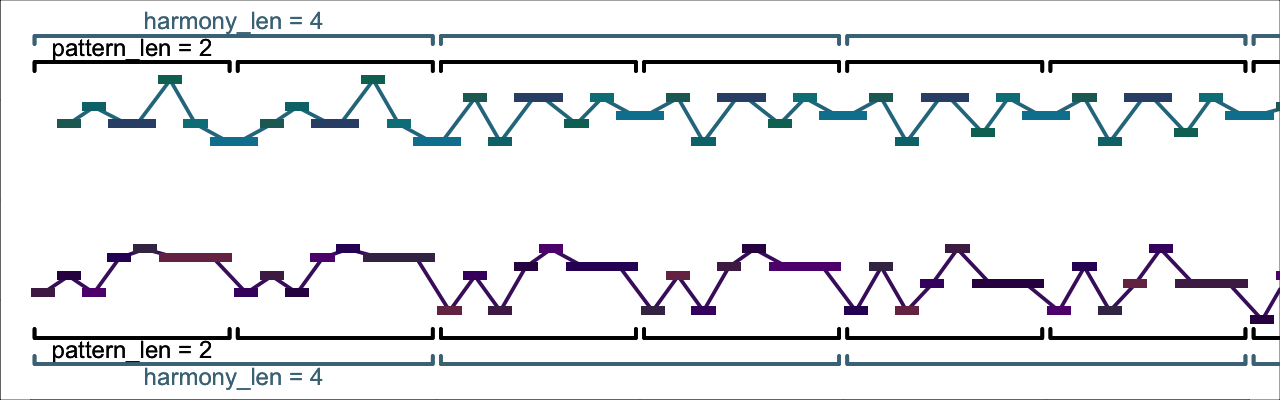Click to open this example in the web app

(By the way, I made the piano-roll figures throughout this documentation with midani.)

Whenever `rhythm_len` is not set explicitly, it is implicitly assigned the value of `pattern_len`. So in the example above, `rhythm_len` was implicitly assigned `2`. In `docs/examples/example2.py`, in contrast, we set `pattern_len = 4`, but `rhythm_len = 2`. Thus, if you look and/or listen carefully, you’ll find that the same rhythm repeats twice on each harmony, but with different notes each time—the entire pattern of pitches takes four beats to repeat, and by the time it does, its pitches are somewhat different, having been adjusted to the new harmony.3

Example: `docs/examples/example2.py` View source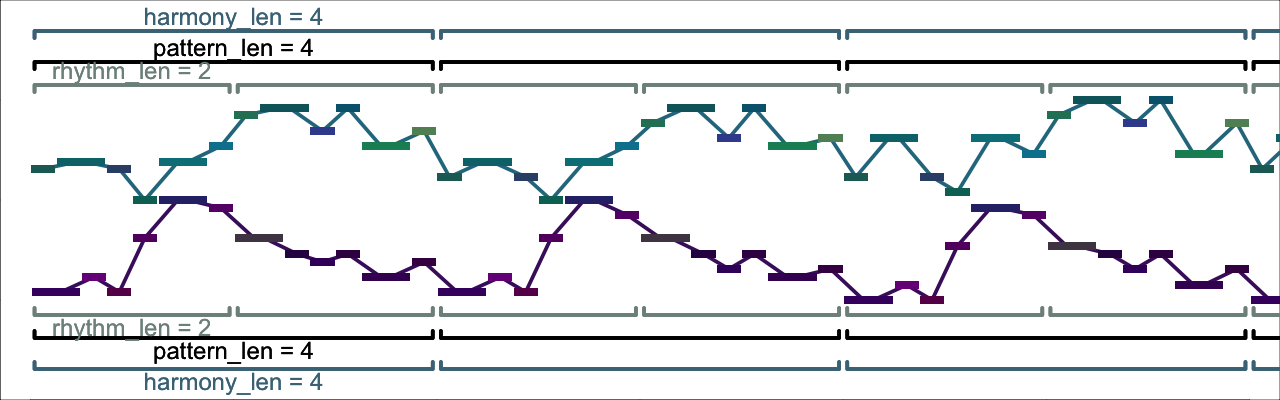Click to open this example in the web app

We aren’t constrained to have `pattern_len` be a whole multiple of `rhythm_len`. In `docs/examples/example3.py`, `pattern_len` is still `4`, but `rhythm_len = 1.5`, so now every third time the rhythm occurs, it is truncated (a bit like a 3–3–2 tresillo pattern).

Example: `docs/examples/example3.py` View source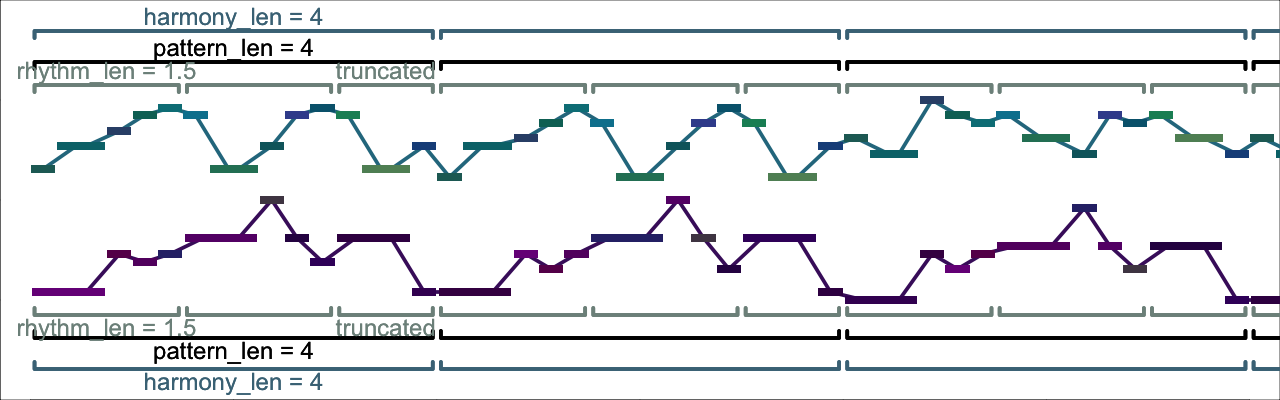Click to open this example in the web app

Up to now, we’ve always specified the same settings in both voices. But we need not do so! In `docs/examples/example4.py`, the bottom voice again has `rhythm_len = 1.5`, but the top voice now has `rhythm_len = 2`.

Example: `docs/examples/example4.py` View source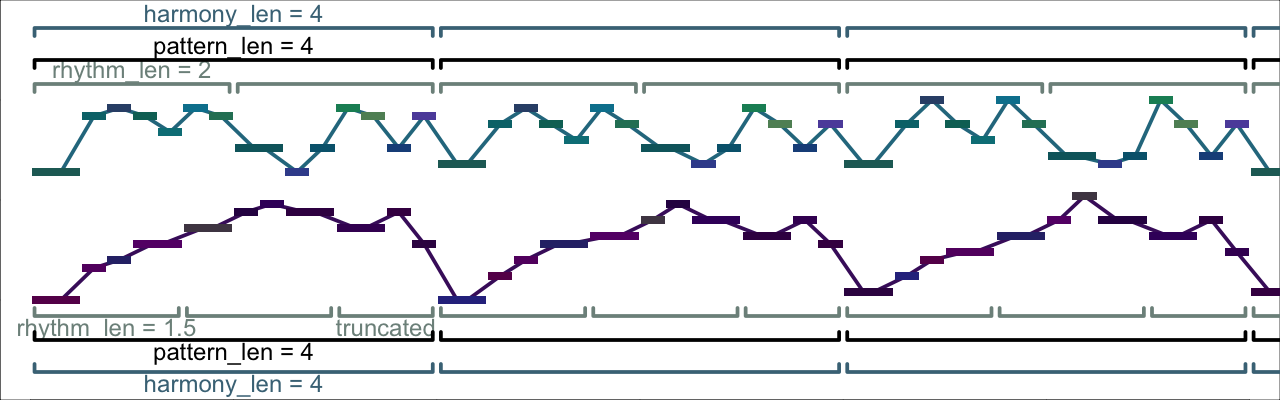Click to open this example in the web app

We can also have different values of `pattern_len` in each voice, as in `docs/examples/example5.py`. However, if we do so, the script has to work quite a bit harder to find a solution. To help it do so, I made its task a little easier by changing `consonance_treatment` from `"all_onsets"` to `"none"`. Thus whereas in the previous examples, the simultaneously onset notes all formed intervals like 3rds and fifths, in `docs/examples/example5.py`, there are also dissonances like 7ths and 9ths.

Example: `docs/examples/example5.py` View source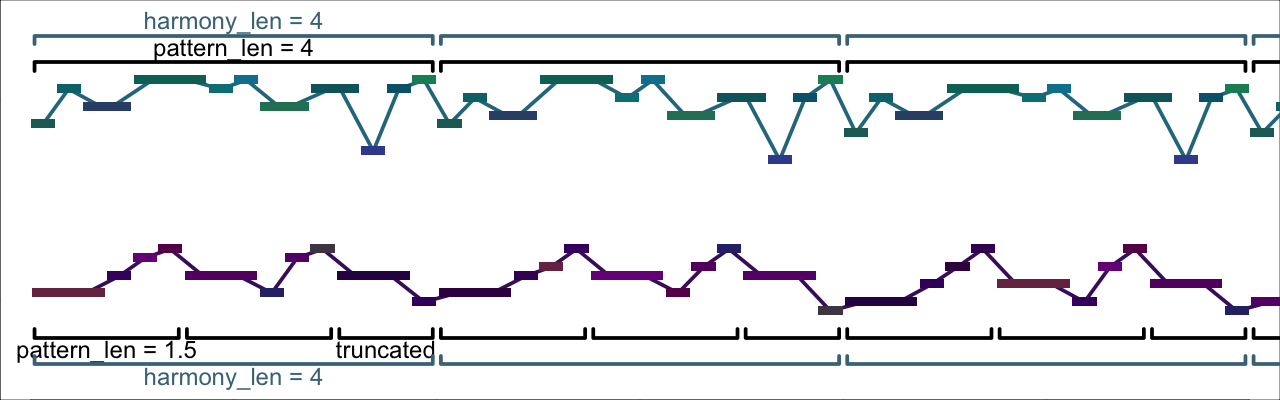Click to open this example in the web app

Up until now, whenever one pattern or rhythm didn’t line up with the other, we have truncated the shorter one, so that subsequent repetitions began together in both voices. But the script doesn’t require this. In `docs/examples/example6.py`, I have changed `truncate_patterns` to `False`. Now the 1.5-beat pattern in the lower part isn’t truncated after 4 beats. Instead, it is displaced relative to both the 4-beat pattern in the upper part, as well as the 4-beat harmony changes. (The two patterns finally come into sync after 12 beats, the least-common-multiple of 1.5 and 4.)

Example: `docs/examples/example6.py` View source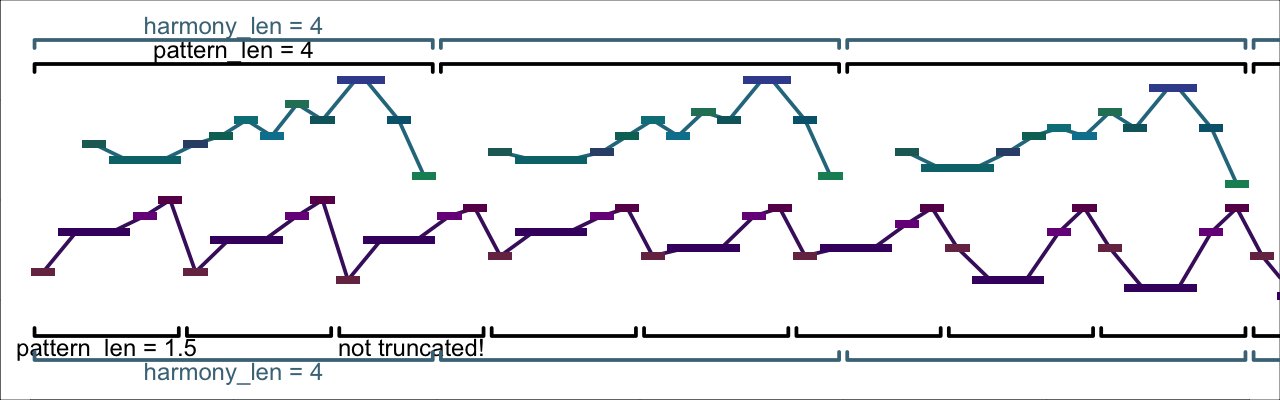Click to open this example in the web app

Another feature of all the examples up to now is that `harmony_len` has always been at least as long as `pattern_len`. But there’s no reason why this has to be so. In `docs/examples/example7.py`, I’ve set `harmony_len = 2` but `pattern_len = 4` so that each pattern covers two harmonies.

Example: `docs/examples/example7.py` View source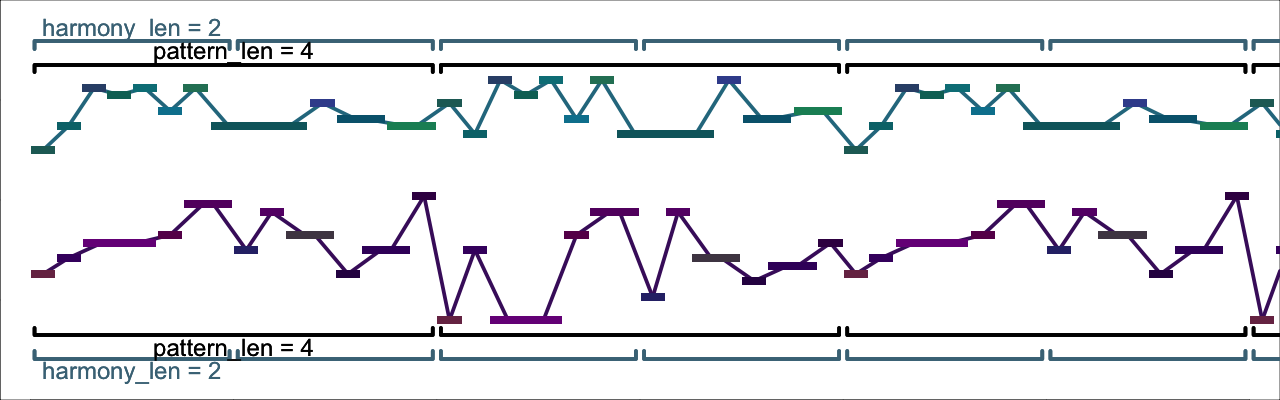Click to open this example in the web app

### Harmony

In the preceding sections, we looked at how to adjust the lengths of patterns, rhythms and harmonies. Now we’ll see how to specify chords and scales to create harmonic progressions.4

The most straightforward way is to specify all chords and scales explicitly. As a first example, I’ve specified one of the most (over?-)used chord progressions in pop music, the I-V-vi-IV progression, in C major. The results are in `docs/examples/harmony_example1.py`. The relevant lines in `harmony_example1.py` are

``````"foot_pcs": ("C", "G", "A", "F"),
"scales": ("MAJOR_SCALE", "MIXOLYDIAN", "AEOLIAN", "LYDIAN"),``````

Example: `docs/examples/harmony_example1.py` View sourceClick to open this example in the web app

There’s a lot to explain here:

1. Strings like `"C"` and `"MAJOR_TRIAD"` name constants that are defined in `src\er_constants.py` and documented here. If you know any music theory, the meaning of the constants above shouldn’t require any further explanation for now.
2. In this script, we call the main bass pitch of each chord its “foot”. The main bass pitch is a little like the “root” of a chord, except that the main bass pitch doesn’t have to be the root of a chord (as in the case of inverted chords).
3. Each foot is associated with the chord and the scale in the same serial position. Both the chord and the scale will be transposed so that they begin on the foot. Thus, there is a one-to-one correspondence between chords and scales (much like the “chord-scale” approach sometimes used in jazz pedagogy).

We can easily put the progression into another key by changing `foot_pcs`. For instance, here it is in E major:

``````"foot_pcs": ("E", "B", "C#", "A"),
"scales": ("MAJOR_SCALE", "MIXOLYDIAN", "AEOLIAN", "LYDIAN"),``````

There are no constraints on `foot_pcs`, so we can get a different progression by changing `foot_pcs` arbitrarily. For example, in `docs/examples/harmony_example2.py` I’ve changed the middle two members of `foot_pcs` to create a more chromatic progression:

``````"foot_pcs": ("E", "G", "D", "A"), # was ("E", "B", "C#", "A")
"scales": ("MAJOR_SCALE", "MIXOLYDIAN", "AEOLIAN", "LYDIAN"),``````

Example: `docs/examples/harmony_example2.py` View source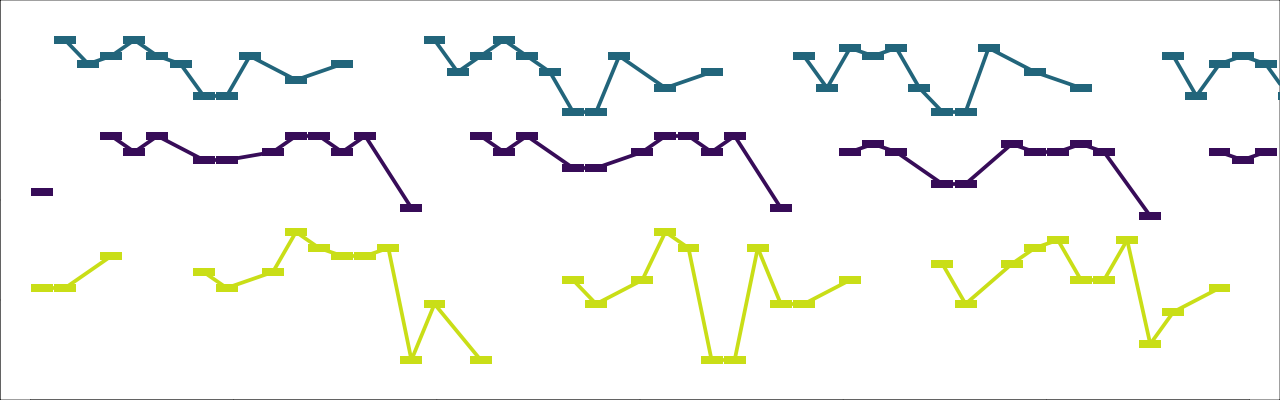Click to open this example in the web app

There are, however, two important constraints on `chords` and `scales`.

1. All the items of `chords` must have the same number of pitch-classes, and all the items of `scales` must as well. This means, for example, you can’t go from a major triad to a seventh chord, or from a major scale to a whole-tone scale. (You can, however, use scales or chords with any number of pitch-classes you like—as long as that number remains the same.)5
2. Every scale must be a superset of the associated chord. So, for example
• `"MAJOR_TRIAD"` will work with `"MAJOR_SCALE"`, `"MIXOLYDIAN"`, or any other scale that contains a major triad beginning on its first pitch
• `"MAJOR_TRIAD"` will not work with `"AEOLIAN"`, `"DORIAN"`, etc., because these scales contain a minor triad beginning on their first pitch

Both `chords` and `scales` will be looped through if they are shorter than `foot_pcs`. `docs/examples/harmony_example3.py` illustrates with the following short loop:

``````    "foot_pcs": ("E", "G"),
"chords": ("MAJOR_TRIAD",), # the trailing commas before the parentheses
"scales": ("MIXOLYDIAN",),  #   are necessary!``````

Example: `docs/examples/harmony_example3.py` View source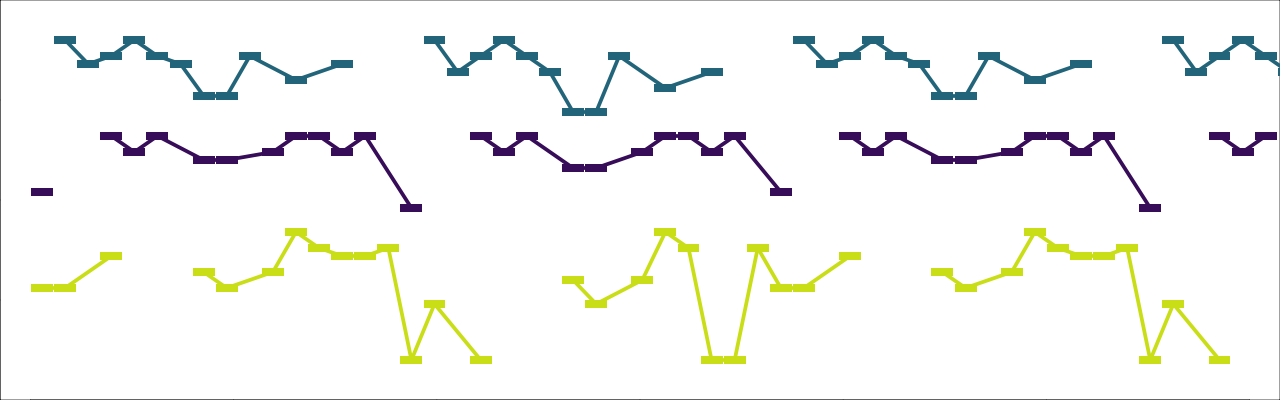Click to open this example in the web app

The sequences `chords` and `scales` do not have to have the same number of items, as `docs/examples/harmony_example4.py` demonstrates:

``````    "foot_pcs": ("E", "G", "E", "C"),
"scales": ("MIXOLYDIAN", "LYDIAN"),``````

Example: `docs/examples/harmony_example4.py` View source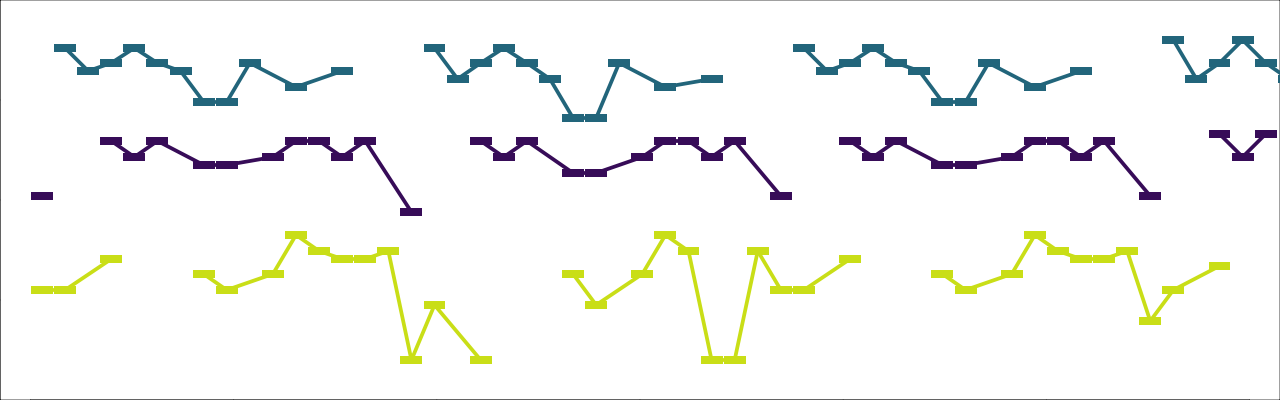Click to open this example in the web app

So far, the length of the progression has always been taken implicitly from the length of `foot_pcs`. But it is also possible to set the length of the progression explicitly, using `num_harmonies`, as in `docs/examples/harmony_example5.py`. Doing so allows us to create “pedal points” on a repeated bass note:

``````    "num_harmonies": 4,
"foot_pcs": ("D",),
# This example also illustrates a strategy for simulating mixing seventh
#   chords with triads, using incomplete seventh chords.
"chords": ("MAJOR_7TH_NO5", "DOMINANT_7TH_NO3", "MAJOR_64", "MAJOR_63"),
"scales": ("MAJOR_SCALE", "MIXOLYDIAN", "DORIAN", "AEOLIAN"),``````

Example: `docs/examples/harmony_example5.py` View source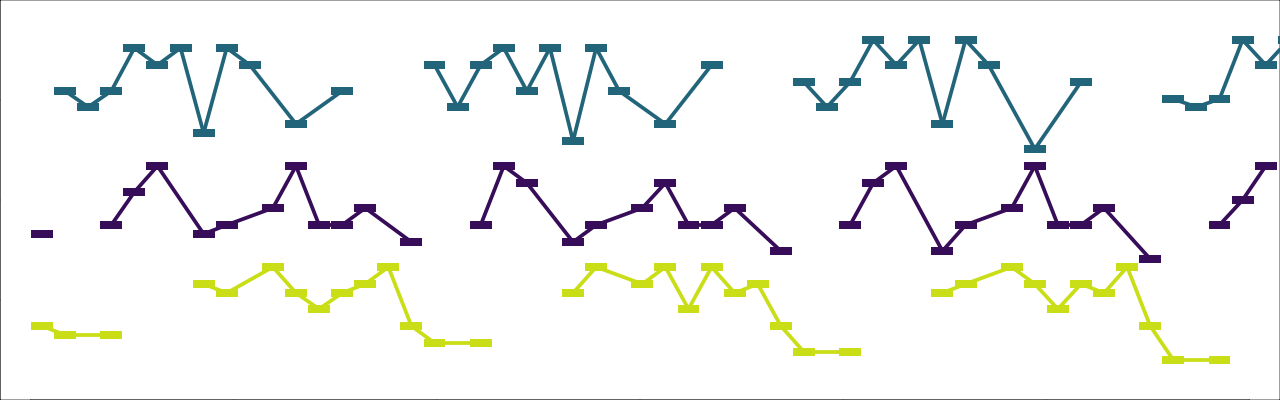Click to open this example in the web app

Another useful setting for creating harmonic progressions is `interval_cycle`. If we pass `interval_cycle` to the script, then any values of `foot_pcs` beyond the first are ignored. Instead, the progression of `foot_pcs` is created by repeatedly progressing upwards by `interval_cycle`. See `docs/examples/harmony_example6.py`:

``````    "num_harmonies": 4,
"interval_cycle": "PERFECT_4TH",
"foot_pcs": ("Eb",),
# The preceding three lines are equivalent to:
#   `"foot_pcs": ("Eb", "Ab", "Db", "Gb")`
"scales": ("MAJOR_SCALE",),``````

Example: `docs/examples/harmony_example6.py` View source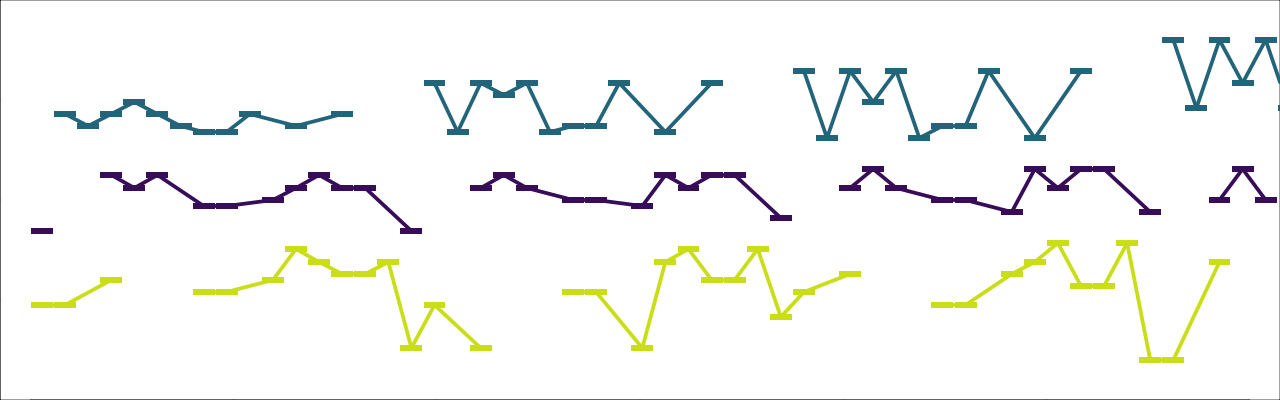Click to open this example in the web app

`interval_cycle` can also consist of more than one interval, as in `docs/examples/harmony_example7.py`. (Note that, since the intervals in `interval_cycle` are always understood upwards, `"MINOR_6TH"` in this example is equivalent to a descending major third.)

``````{
"num_harmonies": 4,
"interval_cycle": ("PERFECT_4TH", "MINOR_6TH"),
"foot_pcs": ("Eb",),
# The preceding three lines are equivalent to:
#   `"foot_pcs": ("Eb", "Ab", "E", "A")`
"scales": ("MAJOR_SCALE",),
}``````

Example: `docs/examples/harmony_example7.py` View source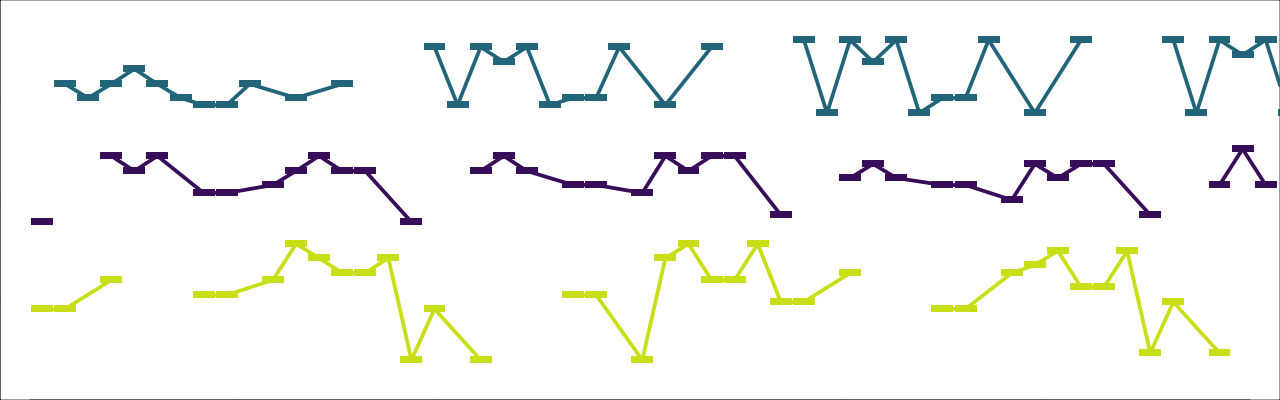Click to open this example in the web app

Before concluding this introduction to specifying harmonies, I should add a few words about what the script actually does with `chords` and `scales`.

• `scales` are used unconditionally: during each harmony, pitches are drawn exclusively from the associated scale. When a pattern is voice-led from one harmony to another, a bijective mapping is effected between the associated scales.
• the use of `chords` is more contingent. The most important relevant settings are

In the preceding examples of this section both `chord_tone_selection` and `voice_lead_chord_tones` have been `True`. As a contrasting illustration, `docs/examples/harmony_example8.py` repeats the settings of `docs/examples/harmony_example6.py`, with the sole difference that `voice_lead_chord_tones` is now set to `False`. (Note, however, that the settings `force_foot_in_bass = True` and `extend_bass_range_for_foots = 7` in `examples/harmony_example_base.py` are still causing the foot of each scale/chord to sound on beat one of each harmony.)

Example: `docs/examples/harmony_example8.py` View source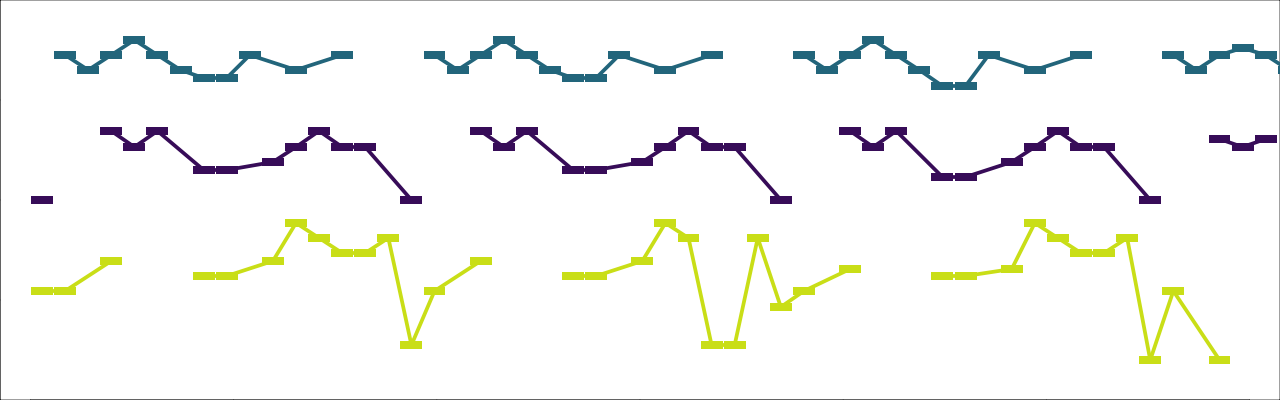Click to open this example in the web app

`docs/examples/harmony_example9.py` is similar, but with `chord_tone_selection = False` as well.

Example: `docs/examples/harmony_example9.py` View source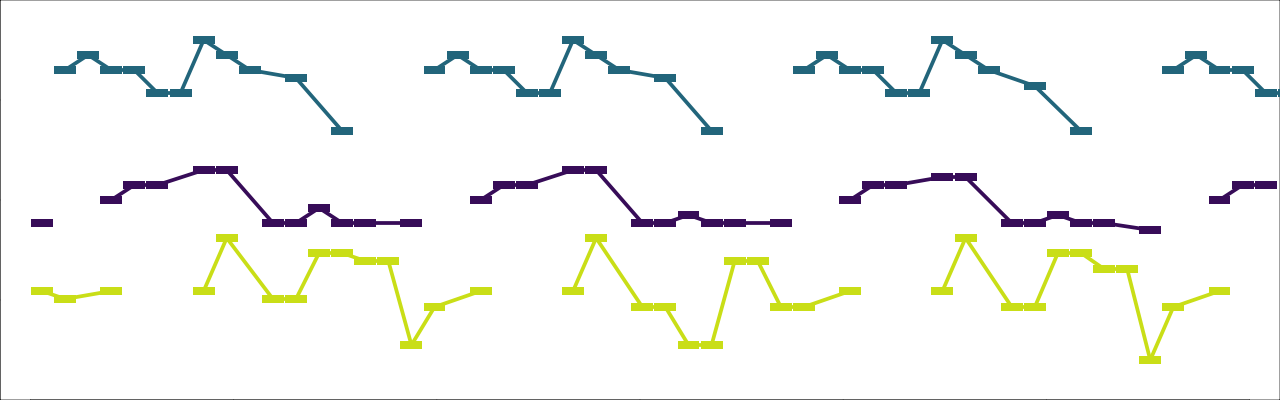Click to open this example in the web app

### Specifying rhythms

Besides setting `rhythm_len` as we did above, there are many other ways of controlling the rhythms that are produced.6

To begin with, we have `onset_subdivision` and `onset_density`:

So for example, in `docs/examples/rhythm_example1.py`, `"onset_subdivision" = 1/4` indicates a sixteenth-note grid, and `"onset_density" = 1.0` indicates that every point in the grid should have an onset, creating a moto perpetuo texture. (For clarity/brevity, this example has only one voice.)

``````{
"num_voices": 1,
"onset_density": 0.5,
"onset_subdivision": 1/4,
}``````

Example: `docs/examples/rhythm_example1.py` View sourceClick to open this example in the web app

If we reduce `onset_density`, as in `docs/examples/rhythm_example2.py`, the proportion of the sixteenth-grid that is filled with onsets will be correspondingly reduced.

``````{
"num_voices": 1,
"onset_density": 0.5,
"onset_subdivision": 1 / 4,
}``````

Example: `docs/examples/rhythm_example2.py` View sourceClick to open this example in the web app

Another important rhythmic parameter is `dur_density`. It specifies the proportion of time that should be filled by note durations, irrespective of how many onsets there are. So far we have left `dur_density` at the default value of `1.0`, which means that all notes last until the next onset in that voice. (In musical terms, all notes are legato.) If we decrease it to 0.75, as in `docs/examples/rhythm_example3.py`, some of the notes will become shorter, so that 75% of the total time of the rhythm is filled by sounding notes.

``````{
"num_voices": 1,
"onset_density": 0.5,
"dur_density": 0.75,
"onset_subdivision": 1 / 4,
}}``````

Example: `docs/examples/rhythm_example3.py` View sourceClick to open this example in the web app

To obtain a staccato effect, we can make `dur_density` still shorter, but to obtain really short notes, we may have to adjust `min_dur` as well, which sets the minimum duration of each pitch as well.

``````{
"num_voices": 1,
"onset_density": 0.5,
"dur_density": 0.25,
"min_dur": 1/8,
"onset_subdivision": 1 / 4,
}``````

Example: `docs/examples/rhythm_example4.py` View sourceClick to open this example in the web app

We can change `"onset_subdivision"` as well. For example, to have a grid of eighth-note triplets, we would set `"onset_subdivision" = 1/3`. And since computers have no problem with precise, strange rhythms, we could also set it to unusual values like `5/13` or `math.pi / 12`.7

``````{
"num_voices": 1,
"onset_density": 0.75,
"dur_density": 0.25,
"min_dur": 1/8,
"onset_subdivision": 3/ 13,
}``````

Example: `docs/examples/rhythm_example5.py` View sourceClick to open this example in the web app

All of the settings we have been looking at so far are “per-voice”, meaning that they can be set to a different value in each voice. If we set `onset_subdivision` to a different unusual value in each voice, we get a particularly chaotic effect. (I find that the chaos can be reined in a bit by setting `rhythm_len` to a short value, creating a brief rhythmic loop.)

``````{
"rhythm_len": 1,
"num_voices": 3,
"onset_density": [0.25, 0.5, 0.75],
"dur_density": [0.25, 0.5, 0.25],
"min_dur": [0.25, 0.25, 1/8],
"onset_subdivision": [3/ 13, 5/12, 6/11],
}``````

Example: `docs/examples/rhythm_example6.py` View source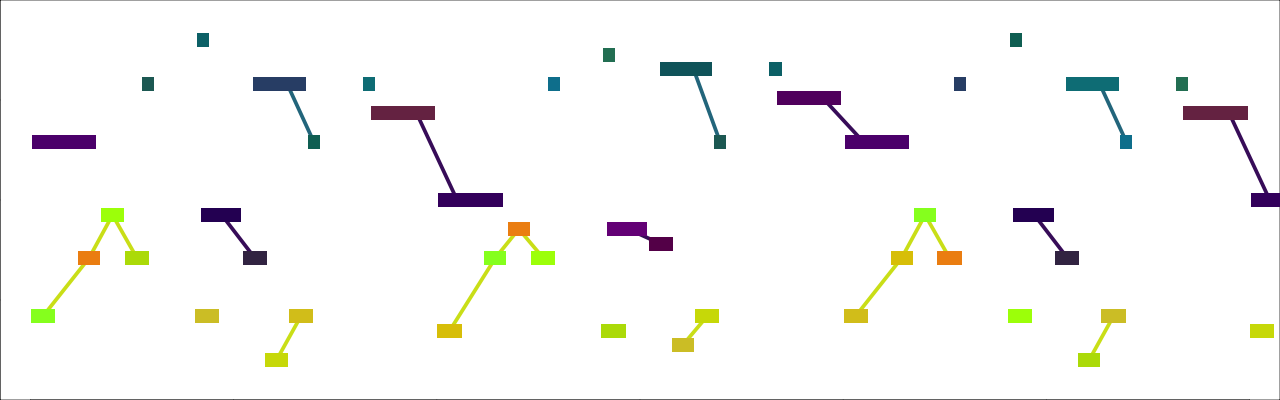Click to open this example in the web app

There are also a few settings that govern the relation between different voices. If `hocketing` is `True`, then, to the extent possible, the onsets of each voice will occur when there is no onset in any other voice. (In textures with many voices, it is also possible to assign specific pairs of voices to hocket with one another.)

``````{
"num_voices": 2,
"onset_density": 0.4,
"dur_density": 0.4,
"min_dur": 0.25,
"onset_subdivision": 0.25,
"hocketing": True,
}``````

Example: `docs/examples/rhythm_example7.py` View source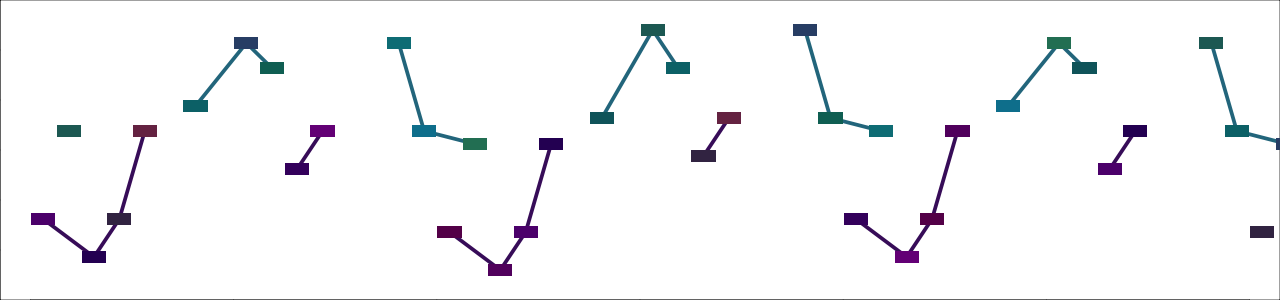Click to open this example in the web app

Another setting that governs the rhythmic relation between voices is `rhythmic_unison`, which causes voices to have exactly the same rhythm. (Like `hocketing`, it can be provided a boolean, or a list of tuples of voices; see the settings documentation for more details.)

``````{
"num_voices": 3,
"rhythmic_unison": True,
"onset_density": .7,
"dur_density": 0.6,
"min_dur": 0.25,
"onset_subdivision": 1/4,
}``````

Example: `docs/examples/rhythm_example8.py` View source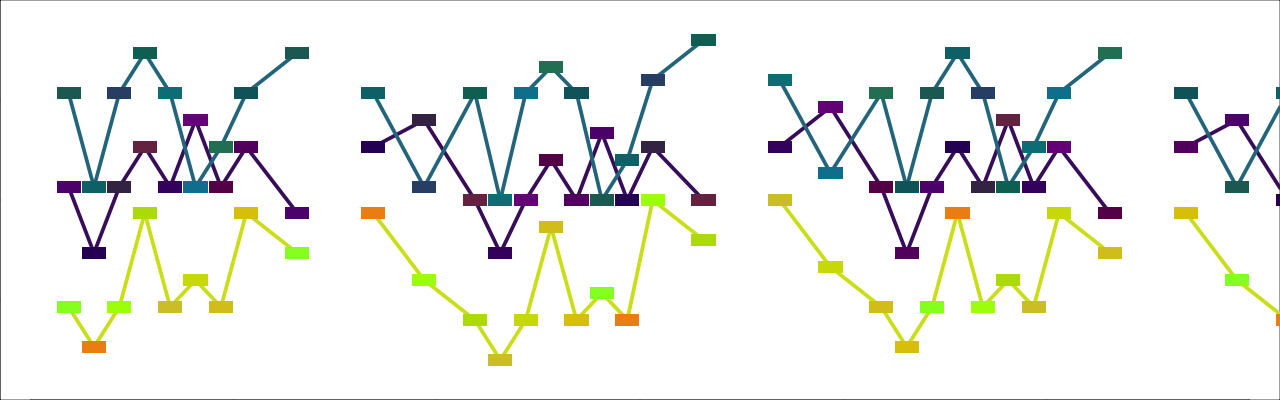Click to open this example in the web app

When `rhythmic_unison` is applied, rhythmic settings like `onset_density` only have any effect in the “leader” voice, whose rhythm is simply copied into the other voices. If we wanted to specify a different `onset_density` in a “follower” voice, it would be ignored. This situation would call for the related setting `rhythmic_quasi_unison`. When `rhythmic_quasi_unison` applies, then, instead of copying the “leader” rhythm into the “follower” voices, the onsets of the “follower” voices are constrained so far as possible to coincide with those of the “leader.” In `docs/examples/rhythm_example9.py`, the leader is the bass voice (midi guitar). The middle voice (midi piano) has a lower `onset_density`, and the top voice (midi electric piano) has a higher `onset_density`.

``````{
"num_voices": 3,
"rhythmic_quasi_unison": True,
"onset_density": [0.5, 0.4, 0.6],
"dur_density": [0.5, 0.4, 0.6],
"min_dur": 0.25,
"onset_subdivision": 1 / 4,
}``````

Example: `docs/examples/rhythm_example9.py` View source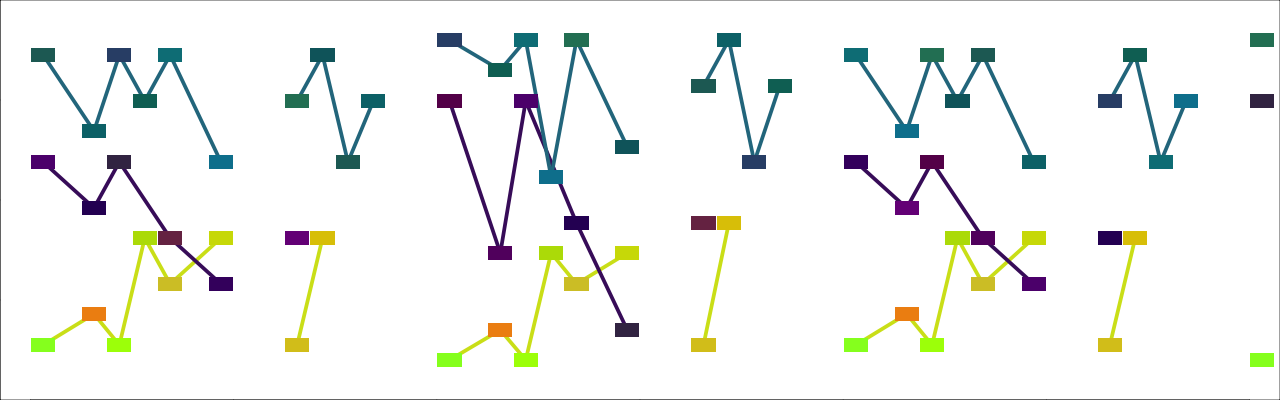Click to open this example in the web app

We can obtain more explicit control of the rhythms through the `obligatory_onsets` setting, which specifies a sequence of times at which the rhythms will be “obliged” to have a note onset. It’s also necessary to specify `obligatory_onsets_modulo` in order to specify when these onsets should repeat (e.g., every two beats).

For example, in `docs/examples/rhythm_example10.py`, I’ve set `obligatory_onsets` to `[0, 0.75, 1.5]` and `obligatory_onsets_modulo` to `2` in order to specify a tresillo 3–3–2 rhythm. Since the value of `onset_density` implies more than three onsets every two beats, additional onsets are added to the underlying scaffold supplied by the values in `obligatory_onsets`.

``````{
"num_voices": 3,
"obligatory_onsets": [0, 0.75, 1.5],
"obligatory_onsets_modulo": 2,
"onset_density": 0.5,
"dur_density": 0.5,
"min_dur": 0.25,
"onset_subdivision": 0.25,
}``````

Example: `docs/examples/rhythm_example10.py` View source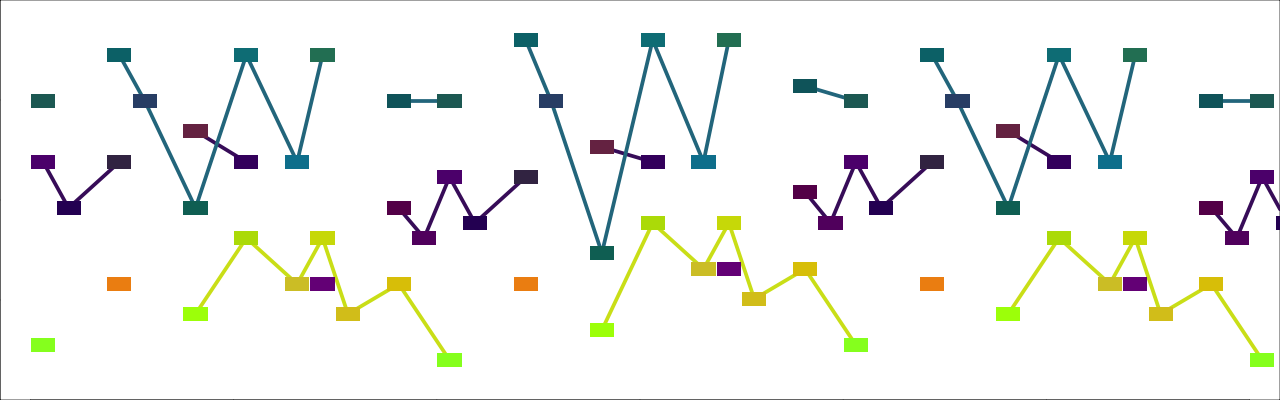Click to open this example in the web app

It is possible to specify an irregular grid upon which note onsets will take place using `sub_subdivisions`. This setting takes a sequence of integers and subdivides the grid specified by `onset_subdivision` into parts defined by the ratio of these integers. For example, in `docs/examples/rhythm_example11.py` below, `sub_subdivisions` is `[4,3]`, which creates an uneven “swing” feel where every first note is 4/3rds as long as every second note.[^To keep the number of total onsets consistent, you’ll probably want to increase `onset_subdivision` by taking the value you otherwise would have chosen and multiplying it by the length of `sub_subdivisions`.] You’ll notice that `docs/examples/rhythm_example11.py` is precisely the same as `docs/examples/rhythm_example1.py`, except for the uneven rhythms.

``````{
"num_voices": 1,
"onset_density": 1.0,
"onset_subdivision": 0.5,
"sub_subdivisions": [4, 3],
}``````

Example: `docs/examples/rhythm_example11.py` View sourceClick to open this example in the web app

I think, however, that it is more interesting to experiment with values of `sub_subdivisions` that are further from what a human would be likely to produce, as I have tried to do in `docs/examples/rhythm_example12.py`.

``````{
"num_voices": 3,
"onset_density": 0.4,
"onset_subdivision": 2,
"sub_subdivisions": [12, 13, 11, 15, 17, 10],
"obligatory_onsets": 0,
"obligatory_onsets_modulo": 2,
"hocketing": True,
}``````

Example: `docs/examples/rhythm_example12.py` View source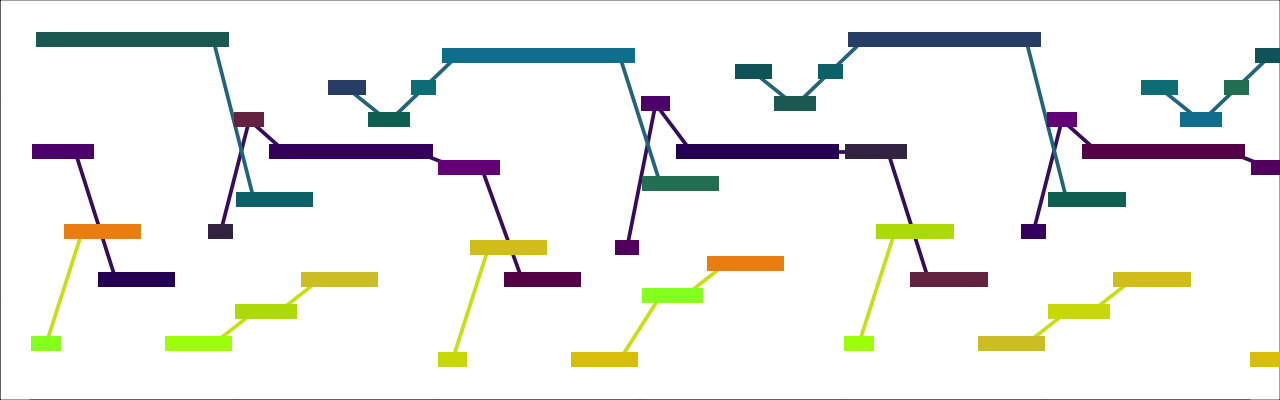Click to open this example in the web app

### Continuous rhythms

What do I mean by “continuous” rhythms? Well, most if not all, human music uses “rational” rhythms, where the durations are (at least notionally) small-integer ratios of one another. For example, a quarter-note is 2 times an eighth-note, 3 times a triplet, 4/3rds of a dotted eighth-note, and so on. But computers don’t need to restrict themselves in this way! We can have a computer make rhythms that arbitrarily chop up the real number line. I have implemented this possibility in this script.8

To better introduce the effect of these continuous rhythms, let’s start with a pattern, without continuous rhythms, that is, with `cont_rhythms = "none"`, its default value. To place a single rhythm as our focus, I’ve set `rhythmic_unison = True`.

Example: `docs/examples/cont_rhythm_example1.py` View source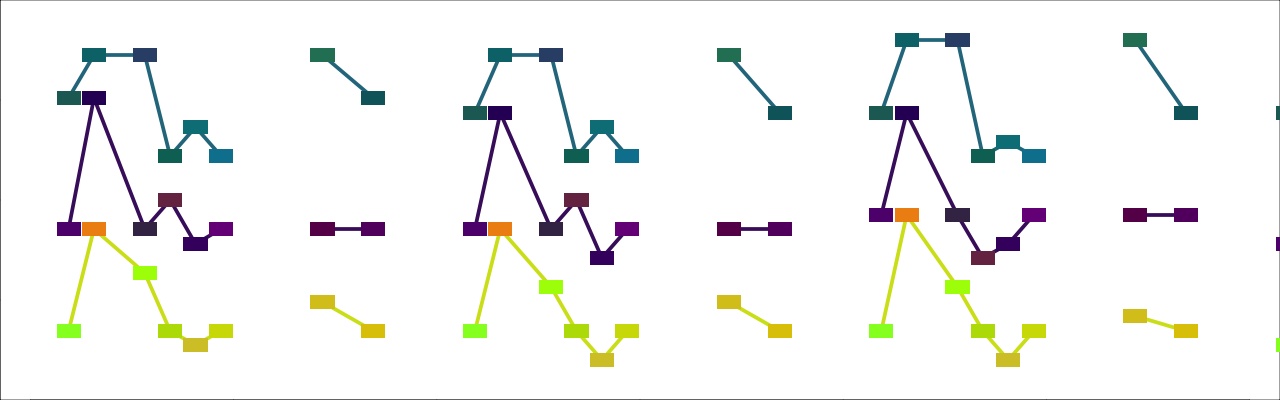Click to open this example in the web app

Now compare the effect of `docs/examples/cont_rhythm_example2.py`, where all the settings are the same, except for `cont_rhythms = "all"`. To me, the rhythm sounds jagged, off-kilter, and sort of incomprehensible, like some sort of alien time signature.

Example: `docs/examples/cont_rhythm_example2.py` View source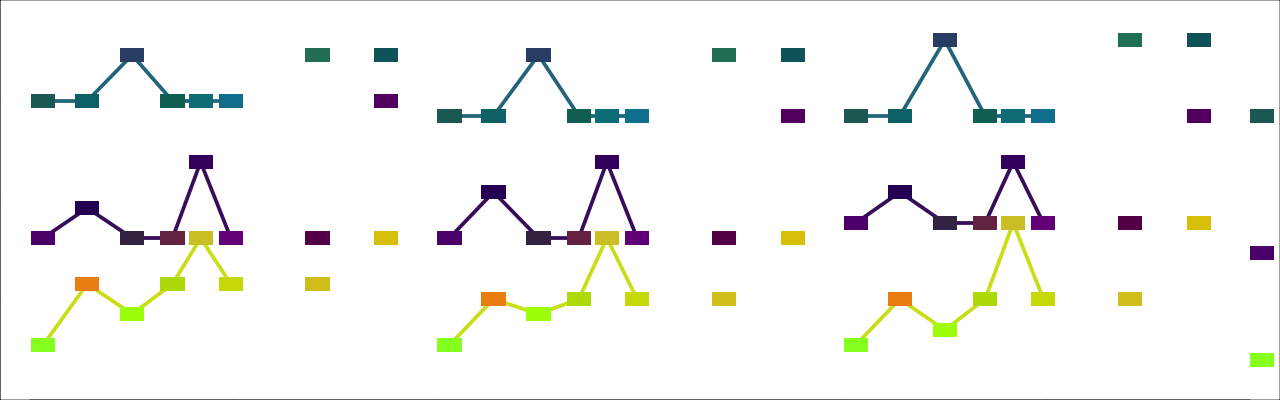Click to open this example in the web app

However, the strangeness of the preceding example is somewhat constrained by the fact that `pattern_len` is not so long that we can’t still readily hear it as a loop. If we set `pattern_len` to a briefer value, like `2`, as in `docs/examples/cont_rhythm_example3.py`, this looping effect is made still stronger, to the extent that the effect of continuous rhythms is fairly subtle.

Example: `docs/examples/cont_rhythm_example3.py` View source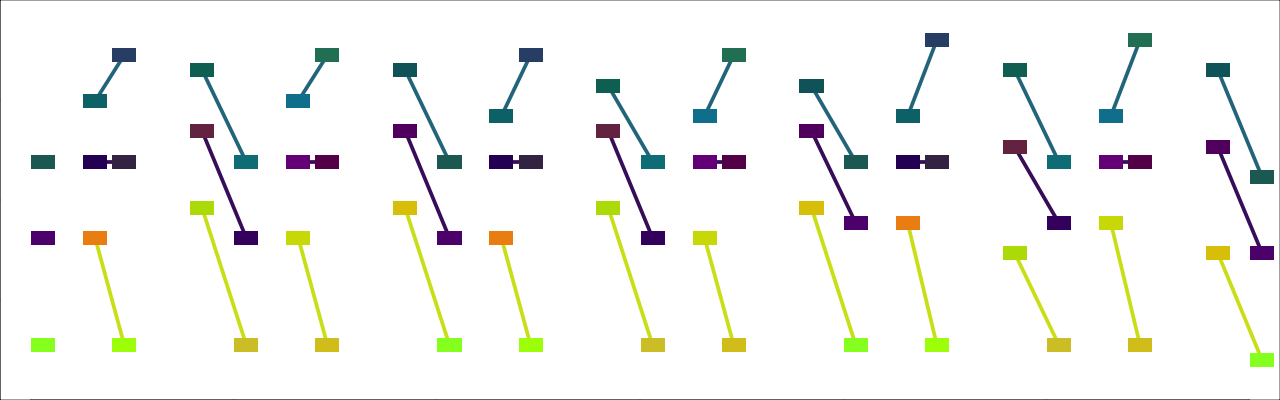Click to open this example in the web app

On the other hand, if we increase pattern_len to `8`, as in `docs/examples/cont_rhythm_example4.py`, the strangeness is increased, and the pattern made less obvious.

Example: `docs/examples/cont_rhythm_example4.py` View source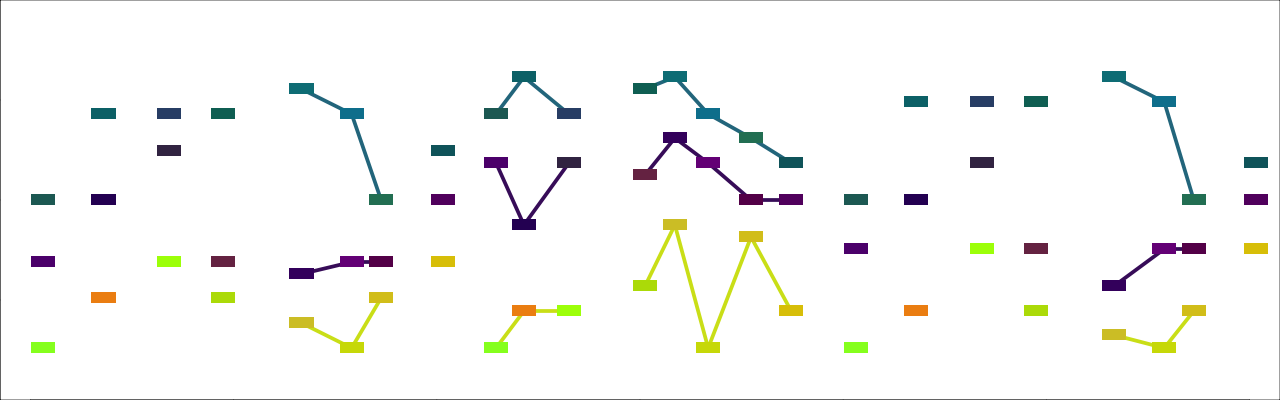Click to open this example in the web app

The number of onsets in a continuous rhythm is calculated as `rhythm_len * onset_density / onset_subdivision`. Thus you can increase the number of notes by increasing `onset_density` or decreasing `onset_subdivision`. The onsets and releases are selected at random from the number line, but this randomness is subject to some constraints. In particular, consecutive note onsets are constrained to be at least `min_dur` from one another. This has the effect of causing the notes to be somewhat evenly spaced, and the larger the value of min_dur, the more evenly spaced they will be, as in `docs/examples/cont_rhythm_example5.py`. `onset_subdivision` / `onset_density` forms an upper bound on min_dur. If `min_dur >= onset_density * onset_subdivision` in a certain voice, then the notes of that voice will be evenly spaced and `cont_rhythms` will have no real effect.9

``````"onset_density": 0.5,
"onset_subdivision": 0.25,
"min_dur": 0.45,``````

Example: `docs/examples/cont_rhythm_example5.py` View source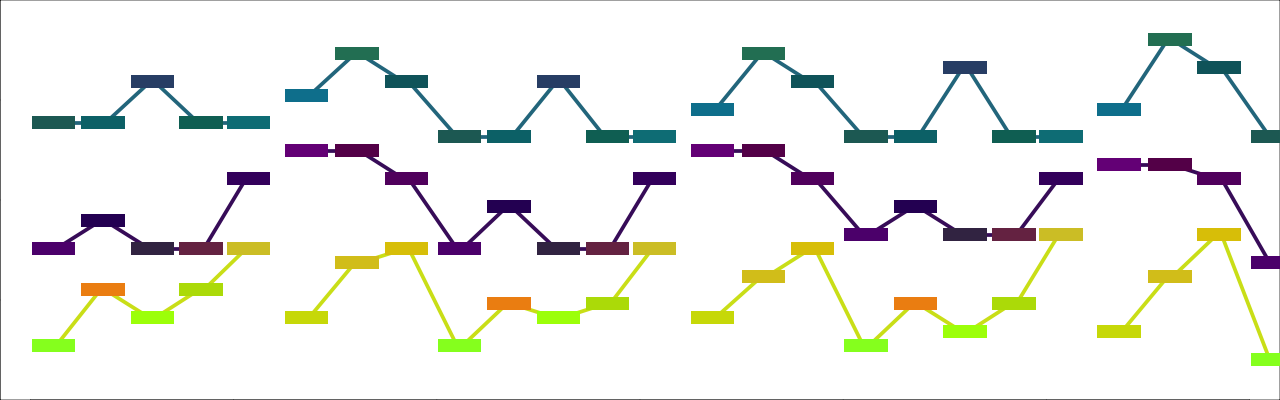Click to open this example in the web app

On the other hand, the more we decrease `min_dur`, the less evenly spaced the notes are constrained to be, and the more they may happen to cluster together randomly. To illustrate, in `docs/examples/cont_rhythm_example6.py`, I’ve reduced `min_dur` from `0.1`, its value in the preceding examples, to `0.01`.

Example: `docs/examples/cont_rhythm_example6.py` View source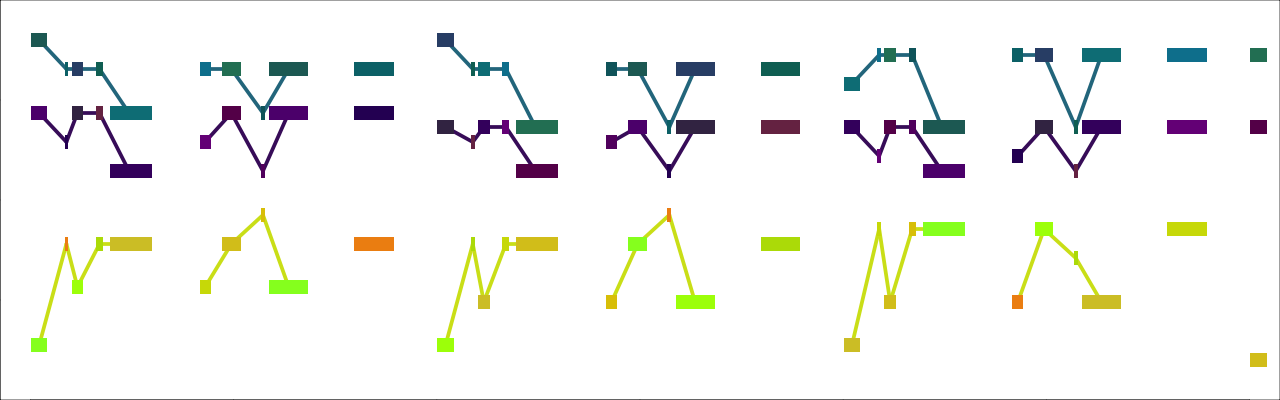Click to open this example in the web app

So far, all the examples have used rhythmic unison. If we set `rhythmic_unison = False`, then each voice will have its own rhythm, as in `docs/examples/cont_rhythm_example7.py`, which has the same settings as `docs/examples/cont_rhythm_example2.py`, except for `rhythmic_unison`. As you can hear, the effect becomes quite chaotic.

Example: `docs/examples/cont_rhythm_example7.py` View source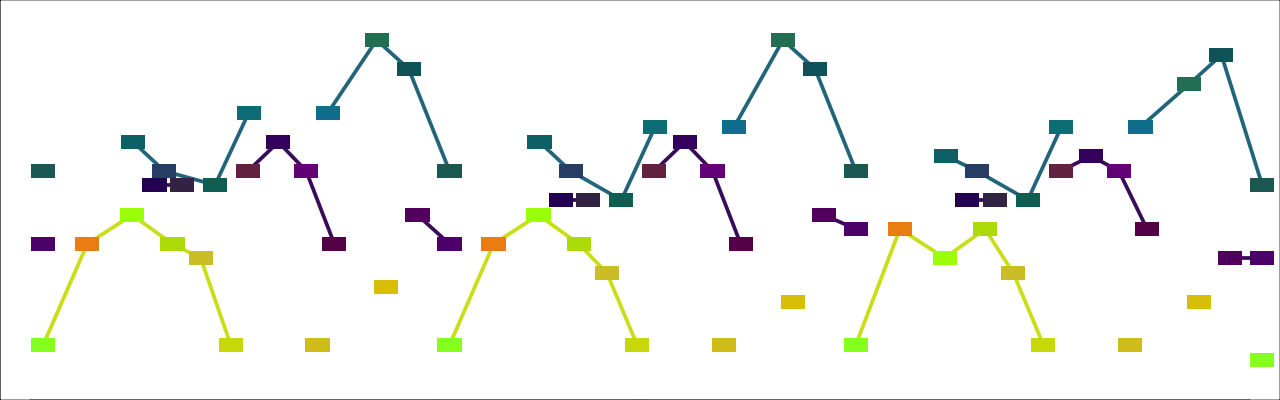Click to open this example in the web app

This chaos emerges because, even though most music features different voices each with their own rhythm, we nevertheless expect the different rhythms to relate to a common metric structure. Here, there is no such common structure—the notes of the various voices seem to occur as random, because they do!

We can reconcile continuous rhythms with a shared metric structure among the different voices by setting `cont_rhythms = 'grid'`. With this setting, the script begins by constructing a continuous metric structure or “grid”, and then creating the rhythms of the various parts by selecting onsets from this grid. You can hear the effect in `docs/examples/cont_rhythm_example8.py`.

Example: `docs/examples/cont_rhythm_example8.py` View source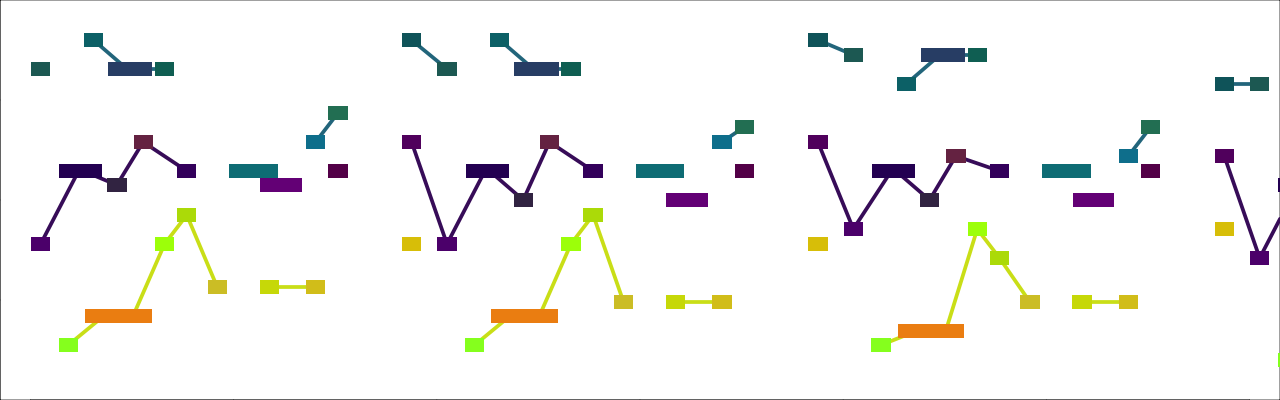Click to open this example in the web app

If `cont_rhythms = 'grid'`, you can also use other rhythmic settings, such as `hocketing`, as in `docs/examples/cont_rhythm_example9.py`.

Example: `docs/examples/cont_rhythm_example9.py` View source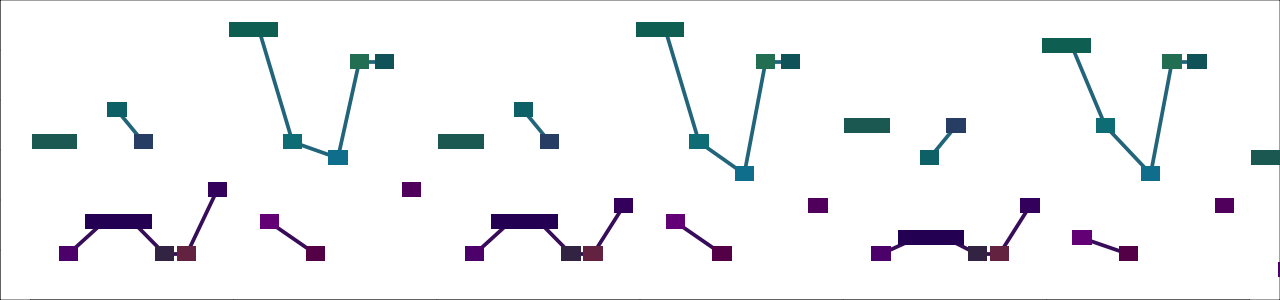Click to open this example in the web app

## Changers: filters and transformers

To make our loops more dynamic and unpredictable, we can use “changers”, functions that take the music produced by the script and filter or transform it in some way.

There are two ways to apply changers:

• The shell script provides an interactive prompt through which changers can be applied and adjusted.
• Changer settings stored in a Python source file can be provided with the `--changers\-c` command-line argument (see the bottom of this section for details).

(Changers are not yet implemented in the web version of Efficient Rhythms.) To illustrate, I will re-use `docs/examples/harmony_example5.py`, already seen above, but repeated just below for your convenience.

Example: `docs/examples/harmony_example5.py` View sourceClick to open this example in the web app

There are two types of changers, “filters,” and “transformers”. Filters remove notes from the score, whereas transformers apply some change to the notes of the score.

The simplest filter is `PitchFilter`, which filters all notes from the score. Of course, we probably don’t want to remove all the notes from the score—we’ll be left with silence. Thus we apply the filter probabilistically. In `docs/examples/changer_example1.py`, the notes are filtered with a probability of 0.5. Thus, (approximately) half of the notes have been removed, and half remain. To my ears, the effect is quite dynamic; the harmonic gist of `docs/examples/harmony_example5.py` remains present, yet in an unpredictable, ever-shifting guise.

Example: `docs/examples/changer_example1.py` View source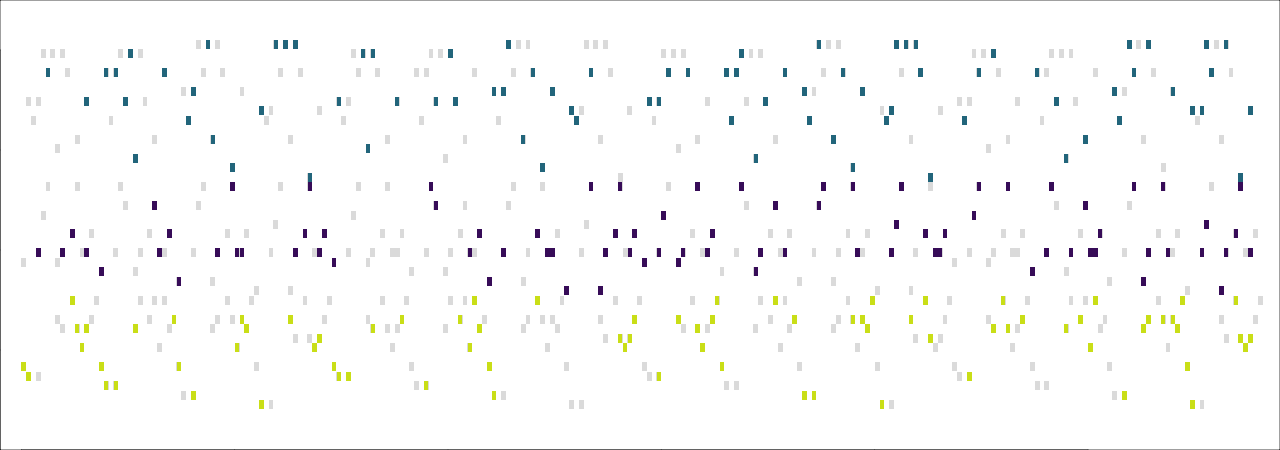This is how the interactive prompt that applies the settings from `docs/examples/changer_example1.py` looks. To save space in subsequent examples, I will show only those settings that have been changed from their default values.

``````## Pitch filter ################################################################
Description: Pitch filter removes notes of any pitch

(1) Probability curve: Static
(2) Segment length range: (1, 1)
(3) Rhythmic granularity: 0
(4) Static probability: 0.5
(5) Only apply to notes marked by:
(6) Start time: 0
(7) End time: 64.0                                 Total length: 64.0
(8) By voice: True
(9) Voices: [0, 1, 2]                          Number of voices: 3
(10) Exemptions: off

We can also apply the filter according to “probability curves”; i.e., functions that take the onset time of a note as argument, and return a probability. In `docs/examples/changer_example2.py`, I apply the filter according to a linear probability curve that decreases steadily from 1.0 to 0.0. Thus, at the start of the score, all of the notes are filtered; by the end, none of them are.

``````## Pitch filter ################################################################
(1) Probability curve: Linear
...
(6) Decreasing: True
...``````

Example: `docs/examples/changer_example2.py` View sourceAlthough each changer can only take a single probability curve, we can include multiple changers, each with its own start and end times, in order to achieve the effect of multiple probability curves. In `docs/examples/changer_example3.py`, I have combined a decreasing curve from the beginning of the score to its middle with an increasing curve from the middle to the end. The effect is that the score begins in silence, emerges in full half way through, and then retreats to silence again.

``````## Pitch filter ################################################################
(1) Probability curve: Linear
...
(6) Decreasing: True
...
(8) Start time: 0.0
(9) End time: 32.0                                 Total length: 64.0
...``````
``````## Pitch filter ################################################################
(1) Probability curve: Linear
...
(6) Decreasing: False
...
(8) Start time: 32.0
(9) End time: 64.0                                 Total length: 64.0
...``````

Example: `docs/examples/changer_example3.py` View source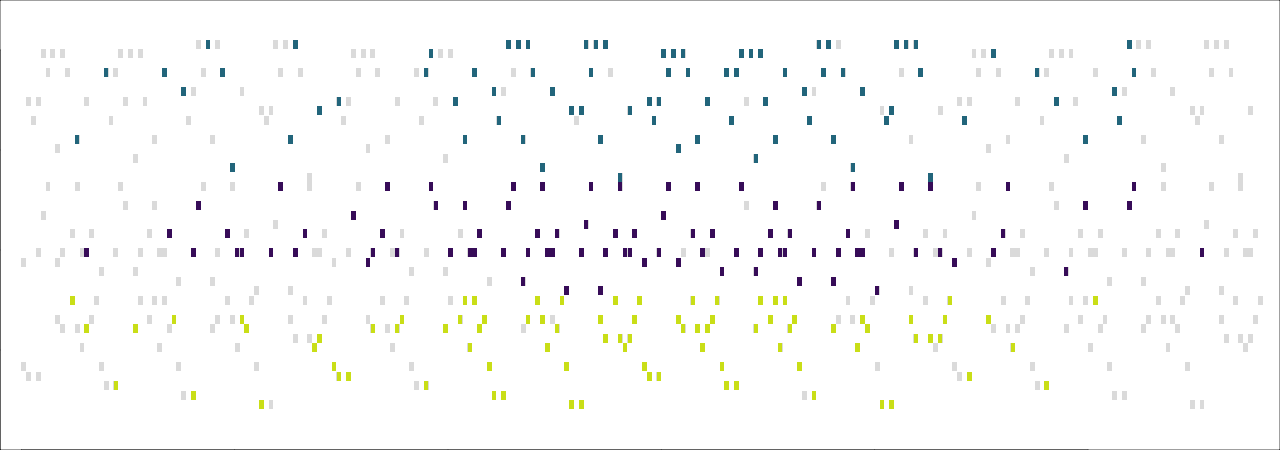If `by_voice` is `True` (its default value), we can apply the filter only to specific voices. We do this by setting `voices` to a list of voice indices. `docs/examples/changer_example4.py` uses the same decreasing pitch filter as `docs/examples/changer_example1.py`, but now the filter applies only to voices 1 and 2. The bass voice (voice 0) is unfiltered and so sounds below the other parts throughout.

``````## Pitch filter ################################################################
(1) Probability curve: Linear
...
(6) Decreasing: True
...
(11) Voices: [1, 2]                             Number of voices: 3
...``````

Example: `docs/examples/changer_example4.py` View source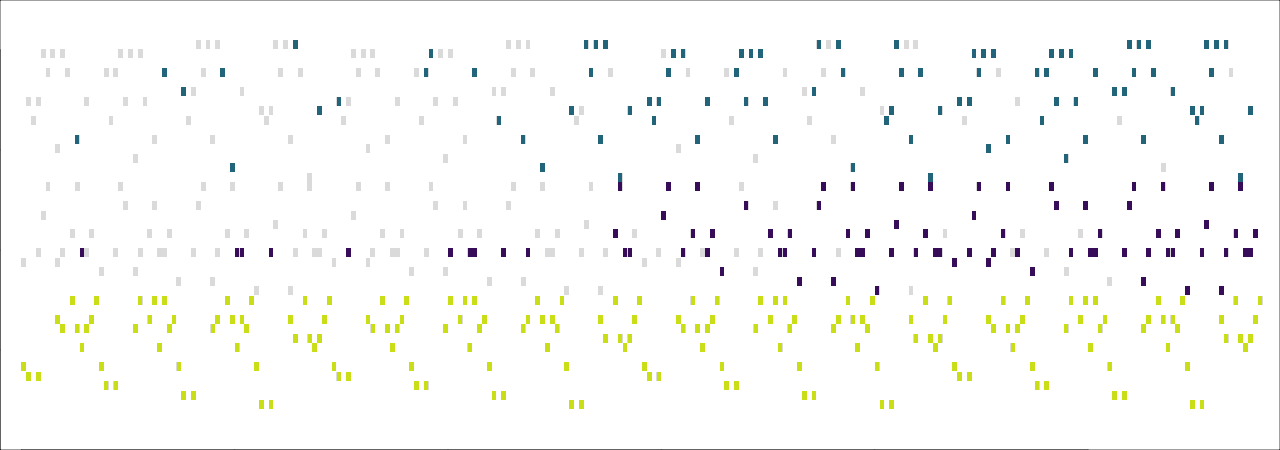On the other hand, if `by_voice` is `False`, then at each onset, all notes in the score sounding at that onset will be filtered as a group. Ref:changer_example5 illustrates by repeating the settings from `docs/examples/changer_example1.py`, but with `by_voice = False`.

``````## Pitch filter ################################################################
(1) Probability curve: Linear
...
(6) Decreasing: True
...
(10) By voice: False
...``````

Example: `docs/examples/changer_example5.py` View source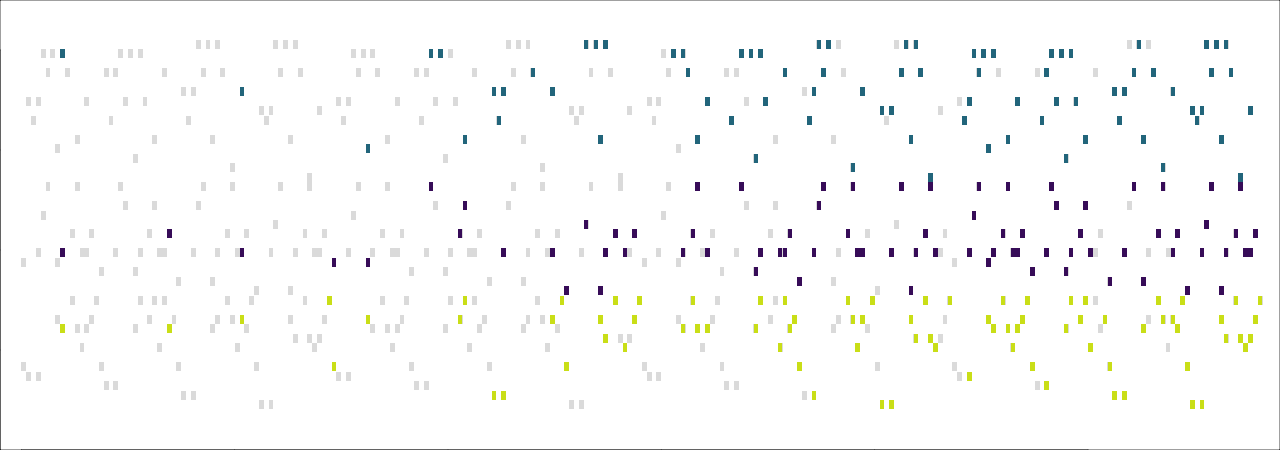Other filters will filter pitches based on some specific condition. For example, `FilterUnselectedPCs` takes a list `selected_pcs` of pitch-classes. The pitch-classes in this list will always pass through the filter, while the remaining pitch-classes will be filtered as normal. This is illustrated in `docs/examples/changer_example6.py`. Since the original music is in the key of D, I have selected pitch classes D and A (= 2 and 9). The effect is that these pitch classes solidly establish the key at the outset, while the other pitches enter gradually like increasingly elaborate embellishments.

``````## Unselected pitch-class filter ###############################################
(1) Probability curve: Linear
...
(6) Decreasing: True
...
(14) Pitch-classes not to filter: [2, 9]                     TET: 12``````

Example: `docs/examples/changer_example6.py` View source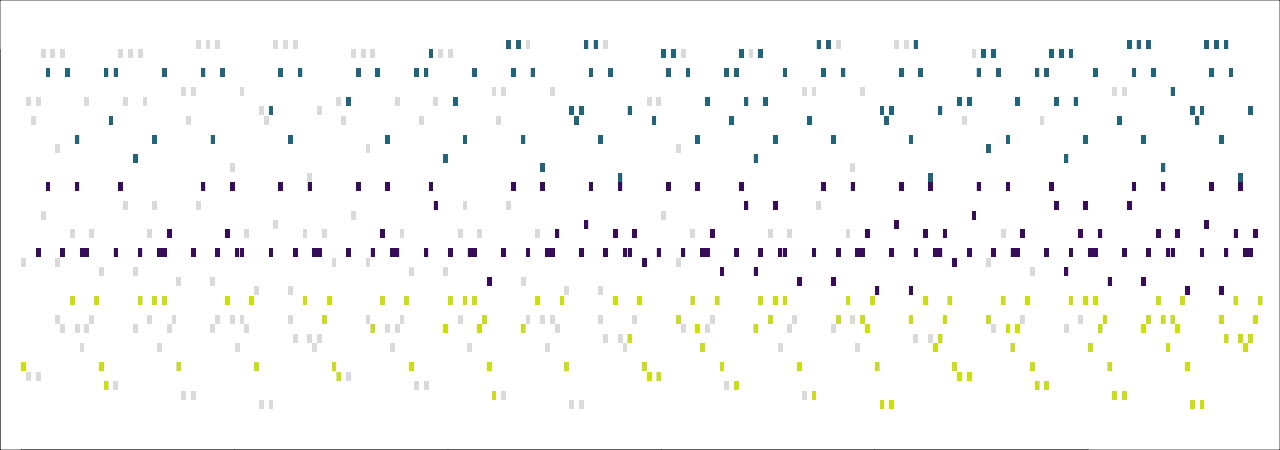A simple example of a transformer is `ForcePitchTransformer`. This transformer takes the argument `force_pitches`, consisting of one or more pitches. It then takes each note and, with probability determined by its probability curve, assigns that note a pitch from `force_pitches`. (If `force_pitches` has more than one element, the choice of pitch is made randomly.) In `docs/examples/changer_example7.py`, I illustrate using pitches 50 (=D3) and 62 (=D4)

``````## Force pitch transformer #####################################################
(1) Probability curve: Linear
...
(6) Decreasing: True
...
(13) Pitches to force: [50, 62]``````

Example: `docs/examples/changer_example7.py` View source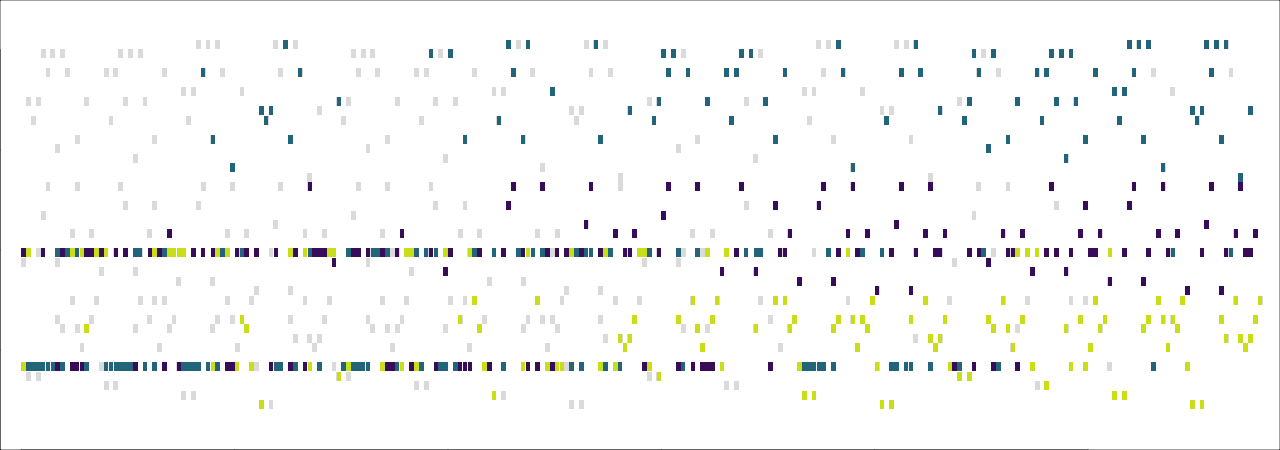Because the music being transformed is in D major, the transformed pitches in the preceding example sound highly consonant. But there’s no requirement that we choose such consonant pitches. As a contrasting example, `docs/examples/changer_example8.py` assigns `force_pitches = [56, 57, 65]` (i.e., G#3, A3, F4). The result is that D major emerges gradually from the ambiguous opening.

``````## Force pitch transformer #####################################################
(1) Probability curve: Linear
...
(6) Decreasing: True
...
(13) Pitches to force: [56, 57, 65]``````

Example: `docs/examples/changer_example8.py` View source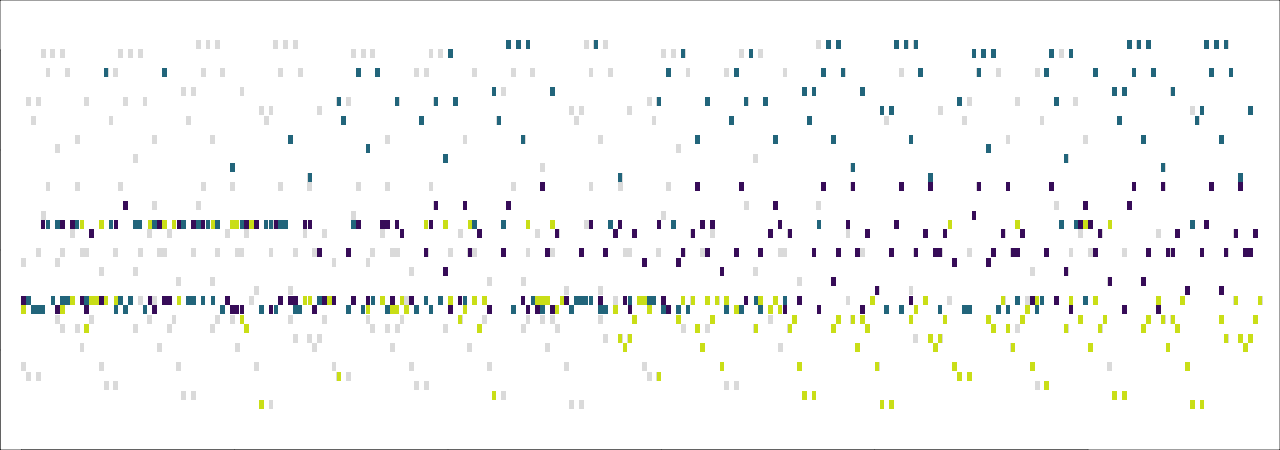There are many other filters and transformers that I have not illustrated here. Preliminary documentation of these changers can be found in changers.html.

If you wish to save your changers using a Python dictionary, the file should consist of a list of 2-tuples. The first item of each tuple is the name of the changer class (e.g., `"PitchFilter"`); the second item is a (possibly empty) dictionary containing the settings for that changer.10 For concrete illustration, see the source files for the above examples.

1. This command needs to be run from the root directory of the repository.↩︎

2. You can find the settings files that generated all the examples in this section in the `docs/examples` folder. `docs/examples/example_base.py` is shared among each example. So you can build the first example with the command `python3 -m efficient_rhythms --settings docs/examples/example_base.py docs/examples/example1.py`; for subsequent examples, just replace `example1.py` with the appropriate file.↩︎

3. The music-theoretically fastidious among you may have observed that these examples contain plentiful parallel fifths (for example, the first two sixteenth-notes in `docs/examples/example2.py`). If desired, parallel fifths could be avoided by including `7` (i.e., the number of semitones in a perfect fifth) in the sequence provided to the setting `prohibit_parallels`.↩︎

4. The settings files that generated the examples in this section all begin with `harmony_example` and are found in the `docs/examples` folder. `docs/examples/harmony_example_base.py` is shared among each example. So you can build the first example with the command `python3 -m efficient_rhythms --settings docs/examples/harmony_example_base.py docs/examples/harmony_example1.py`.↩︎

5. There is a technical reason for this constraint, (namely, that the script works by finding bijective voice-leadings between chords and scales), but in the longterm, I would very much like to remove it.↩︎

6. The settings files that generated the examples in this section all begin with `rhythm_example` and are found in the `docs/examples` folder. `docs/examples/rhythm_example_base.py` is shared among each example. So you can build the first example with the command `python3 -m efficient_rhythms --settings docs/examples/rhythm_example_base.py docs/examples/rhythm_example1.py`.↩︎

7. However, we’ll have to give up on representing these in conventional music notation. In fact, for the time being only duple note-values (i.e., eighth-notes, quarter-notes, and the like) can be exported to notation.↩︎

8. Of course, unless you have invented an infinite precision computer, the rhythms aren’t truly drawn from the continuous real number line.↩︎

9. In general, you probably won’t want this to occur: if you’ve set `cont_rhythms` to a value other than `'none'`, you probably want it to have an effect. But as a special effect, one can have the notes evenly spaced in one voice, while `cont_rhythms` is allowed to wreak havoc in the other voices.↩︎

10. If you’re wondering why a list of 2-tuples, rather than a dictionary, it is to allow for more than one changer of the same class.↩︎